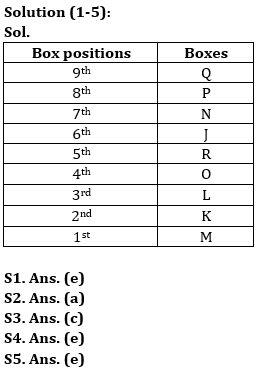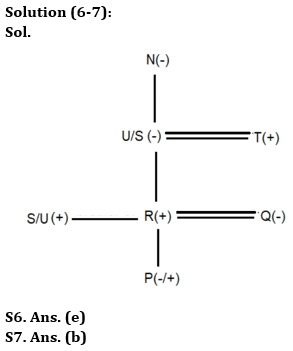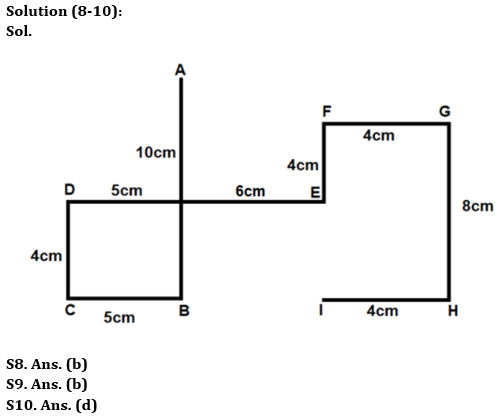Latest Banking jobs   »

# Reasoning Ability Quiz For Bank Foundation 2023 -17th May

Directions (1-5): Answer the questions based on the information given below.
Nine boxes (J, K, L, M, N, O, P, Q, and R) are kept one above the other, but not necessarily in the same order. The bottommost box is numbered as 1 and the topmost box is numbered as 9.
N is kept 3rd above of O. O is 4th box. P which is a perfect cube numbered box (but not 1), is kept immediately below Q. Two boxes are kept between L and J. J is kept adjacent to N. M is kept immediately below K.

Q1. Which of the following is 2nd box?
(a) M
(b) Q
(c) R
(d) L
(e) None of the above

Q2. R is kept ___________ below of Q.
(a) 4th
(b) 2nd
(c) 3rd
(d) 5th
(e) None of the above

Q3. Which box is kept immediately above L?
(a) K
(b) M
(c) O
(d) R
(e) None of the above

Q4. How many boxes are kept below R?
(a) Five
(b) Two
(c) One
(d) None
(e) None of the above

Q5. Which of the following is not a prime numbered box?
(a) N
(b) R
(c) K
(d) L
(e) J

Directions (6-7): Answer the questions based on the information given below.
There are seven members in a family of four generations. T is father of R’s brother. Q is mother of P. N is mother-in-law of T. R is married. The genders of S and U are opposite to each other. Q is not married to T.

Q6. How is S related to Q?
(a) Father-in-law
(b) Brother-in-law
(c) Son-in-law
(d) Mother-in-law
(e) Can’t be determined

Q7. How is R related to N?
(a) Grandfather
(b) Grandson
(c) Sister
(d) Mother
(e) None of these

Directions (8-10): Answer the questions based on the information given below.
There are nine points A, B, C, D, E, F, G, H and I, drawn on a sheet of paper. B is 10cm to the south of A. D is 11cm to the west of E. D is 4cm to the north of C. G is 4cm to the east of F. H is 8cm to the south of G.I is 4cm to the west of H. C is 5cm to the west of B. F is 4cm to the north of E.

Q8. What is the direction of point A with respect to point I?
(a) North-East
(b) North-west
(c) North
(d) South-west
(e) None of these

Q9. What is the shortest distance between point C and point H?
(a) 13m
(b) 15m
(c) 12m
(d) 18m
(e) None of these

Q10. What is the direction of point C with respect to point G?
(a) North-East
(b) North-west
(c) South-east
(d) South-west
(e) None of these

Solutions## FAQs

### What is the selection process of the Bank Clerk?

The selection process of the Bank Clerk is Prelims & Mains.

#### Congratulations!Union Budget 2023-24: Free PDF